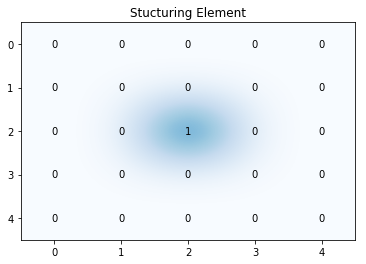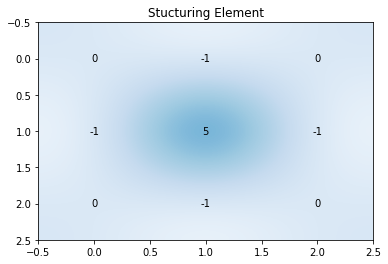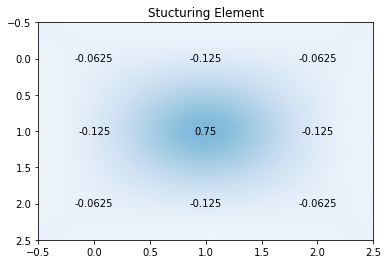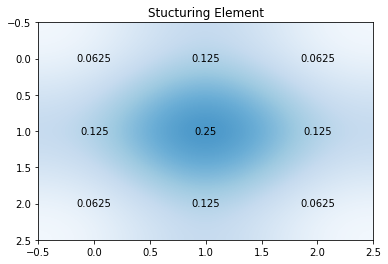Notebook

# Digital Image Processing

In :
% run load_lib_ch4.py


## Chapter 4: Linear Image Processing

Unlike morphological processing, linear processing relies on the pixel intensities in the neighbourhood around a given pixel (x,y). This is done by convolution. A kernel matrix of values is applied on each pixel. Linear processing is used for various purposes such as sharpening, filtering and blurring.

### Typical kernels and their functions

• Identity kernel: Makes no change. Defined as a kernel of zeros with a single one at the centre.
In :
kernel = np.array([
[0,0,0,0,0],
[0,0,0,0,0],
[0,0,1,0,0],
[0,0,0,0,0],
[0,0,0,0,0]
], dtype=np.uint8)

In :
convolutional_element_plot(kernel)In :
compare_result(img, cv2.filter2D(img,-1,kernel))• Sharpening: Makes the image appear sharper.
In :
kernel = np.array([
[0,-1,0],
[-1,5,-1],
[0,-1,0]
], dtype=np.int8)

In :
convolutional_element_plot(kernel)In :
compare_result(img, cv2.filter2D(img,-1,kernel))• High pass filter: Alternative way to sharpen an image. Defined as identity kernel minus a gaussian kernel (also described in this chapter). Applying this filter shows the high intensity pixels of the image. It is then summed with the original image to enhance those pixels.
In :
kernel = np.array([
[-1,-2,-1],
[-2,12,-2],
[-1,-2,-1]
], dtype=np.int8)/16

In :
convolutional_element_plot(kernel)In :
scaling_factor=2.5• Gaussian Blurring

An effective kernel to blur a digital image is often based on the Gaussian function for 2D, $G(x,y)={\frac {1}{2\pi \sigma ^{2}}}e^{-{\frac {x^{2}+y^{2}}{2\sigma ^{2}}}}}$. However this function is continuous and can only be applied on analogue images. In order to use it digitally, an approximation kernel is made. An approximation can be made using Pascal's pyramid. For a kernel with size $n$ x $n$ and Pascal pyramid line $P_n$. The kernel is symmetrical and weighted by dividing by the sum of all it's members.

$K_{n.n} = [P_n]* \begin{bmatrix} P_n \\ P_n \\ ... \\ P_n \end{bmatrix}.\cfrac{1}{sum}$

In :
kernel = np.array([
[1,2,1],
[2,4,2],
[1,2,1]
], dtype=np.uint8)/16

In :
convolutional_element_plot(kernel)In :
compare_result(img, cv2.filter2D(img,-1,kernel))The blur is more noticable when a larger sized kernel is used:

In :
compare_result(img, cv2.GaussianBlur(img,(9,9),0))